# MCAT Physical : Oxidation-Reduction in Electrochemistry

## Example Questions

### Example Question #1 : Oxidation Reduction In Electrochemistry

Imagine a galvanic cell that uses solid zinc and aqueous iron ions to produce a voltage.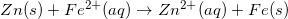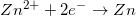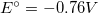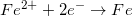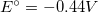Based on the above reaction, which of the following statements is correct?is reduced at the cathode, and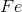is oxidized at the anode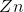is oxidized at the anode, and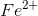is reduced at the cathodeis reduced at the cathode, andis oxidized at the anodeis oxidized at the cathode, andis reduced at the anodeis reduced at the anode, andis oxidized at the cathodeis oxidized at the anode, andis reduced at the cathode

Explanation:

For the MCAT, remember "ANOX REDCAT." This will remind you that oxidation occurs at the anode, and reduction occurs at the cathode.

Since solid zinc (Zn) is giving up two electrons in the reaction, it is being oxidized. The iron ion (Fe2+) is accepting the two electrons, so it is being reduced.

### Example Question #2 : Oxidation Reduction In Electrochemistry

Which of the following is false about anodes and cathodes?

I. In a galvanic cell only, reduction occurs at the anode whereas oxidation occurs at the cathode

II. Electron flows from anode to cathode in both galvanic and electrolytic cells

III. Cathodes are only found in galvanic cells

I and III

III

I, II, and III

II and III

I and III

Explanation:

An electrochemical cell undergoes two half-cell reactions (one oxidation reaction and one reduction reaction). Each half-cell reaction occurs either in a cathode or an anode; therefore, an electrochemical cell always has a cathode and an anode. Recall that there are two types of electrochemical cells: a galvanic cell and an electrolytic cell. Regardless of the type of the electrochemical cell, the reduction half-cell reaction always occurs in the cathode and the oxidation half-cell reaction always occurs in the anode; therefore, both galvanic and electrolytic cells have reduction occurring in the cathode and oxidation occurring in the anode.

In both galvanic and electrolytic cells, electrons flow from the anode to the cathode. Anodes have free electrons because electrons are products of an oxidation reaction whereas cathodes require electrons because electrons are reactants of a reduction reaction; therefore, the free electrons flow from the anode to the cathode where they are used up in the reduction reaction.

Cathodes are found in both galvanic and electrolytic cells. Recall the cathodes are the site of reduction half-reaction and anodes are the site of oxidation half-reaction.

### Example Question #3 : Oxidation Reduction In Electrochemistry

Electrons are __________ in cathodes and __________ in anodes.

products . . . products

reactants . . . products

products . . . reactants

reactants . . . reactants

reactants . . . products

Explanation:

The cathode is the site of reduction half-reaction, whereas the anode is the site of the oxidation half-reaction. In a reduction reaction molecules acquire electrons, whereas in an oxidation reaction molecules lose electrons. Electrons act as reactants during reduction reactions in the cathode, whereas they serve as products during oxidation reactions in the anode.

### Example Question #4 : Oxidation Reduction In Electrochemistry

Consider the reaction below: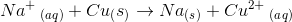The sodium half-cell is found at the anode because sodium is being oxidized

The half-cell locations cannot be determined without knowing the electrochemical cell potential of the overall reaction

The sodium half-cell is found at the cathode because sodium is being oxidized

The sodium half-cell is found at the cathode because sodium is being reduced

The sodium half-cell is found at the cathode because sodium is being reduced

Explanation:

In the reaction, a sodium ion (with a charge of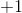) becomes a neutral sodium metal. This means that the sodium ion gained an electron and was reduced in the reaction. On the other hand, copper metal went from a neutral molecule to a copper ion with a charge of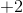; therefore, copper metal lost two electrons and was oxidized in the reaction. Recall that the reduction half-reaction always occurs in the cathode and the oxidation half-reaction always occurs in the anode. This means that the sodium half-cell will be found at the cathode and the copper half-cell will be found at the anode.

To solve this question, we don’t need to know the electrochemical cell potential of the reaction. The electrochemical cell potential signifies whether the reaction is spontaneous (positive cell potential) or nonspontaneous (negative cell potential). A spontaneous reaction will occur in a galvanic cell and a nonspontaneous reaction will occur in an electrolytic cell. Remember that, regardless of the type of electrochemical cell, the reduction half-reaction always occurs at the cathode and the oxidation half-reaction always occurs at the anode; therefore, in both cells, the sodium half-cell will be in the cathode and the copper half-cell will be in the anode.

### Example Question #5 : Oxidation Reduction In Electrochemistry

Which of the following is the correct direction of electron flow in an electrochemical cell?

Cathode to anode

The electron flow direction depends on the type of electrochemical cell

Anode to cathode

The electron flow direction depends on the metals involved in the half-reactions